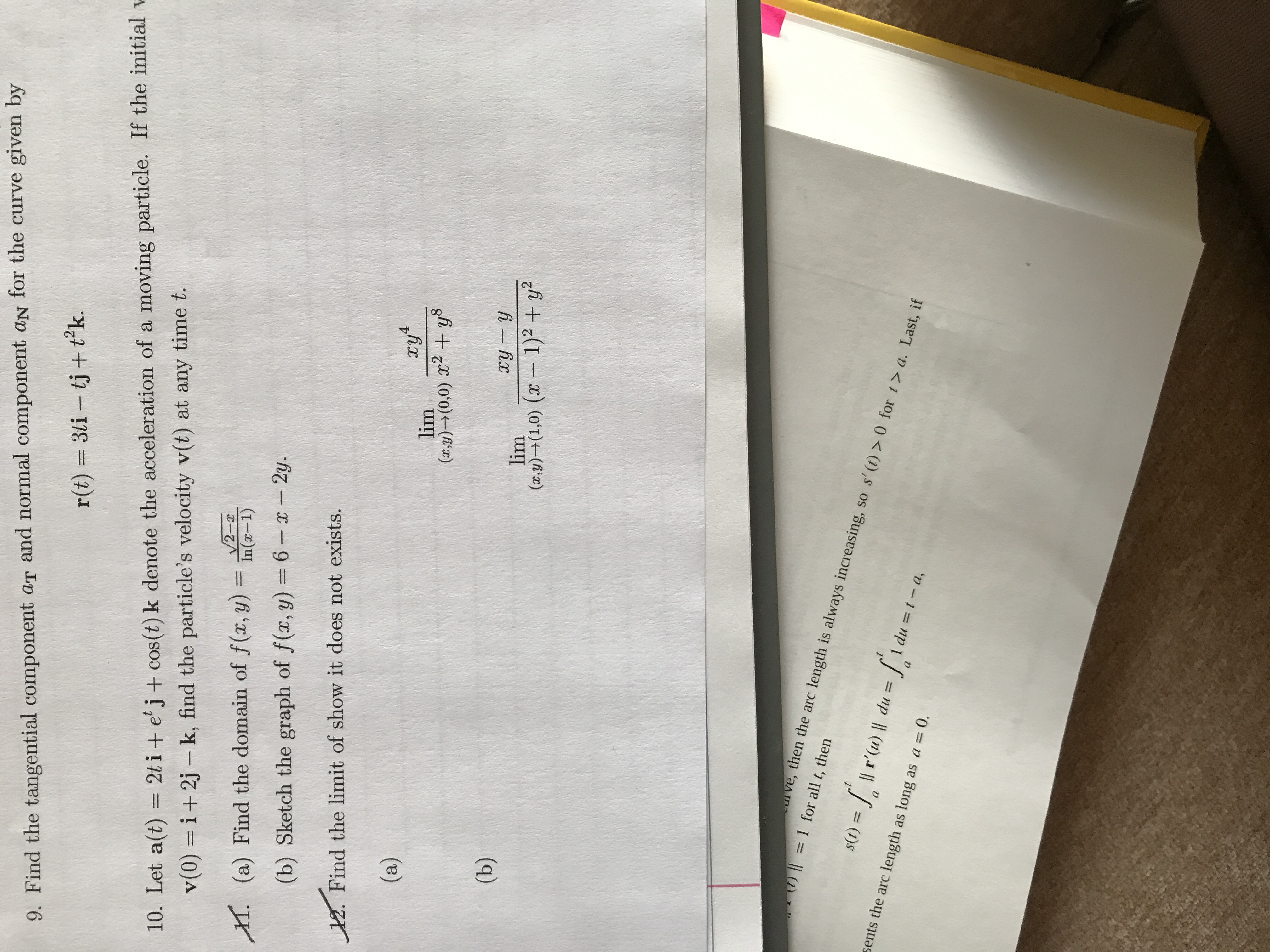# 9. Find the tangential component aT and normal component aN for the curve given byr(t) 3ti - tj + tk.10. Let a(t) 2ti + e'j+ cos (t) k denote the acceleration of a moving particle. If the initialv(0)= i+2j- k, find the particle's velocity v(t) at any time t.V2-xIn(-1)(a) Find the domain of f (x, y)H(b) Sketch the graph of f(x, y) = 6-x-2y.Find the limit of show it does not exists.4(a)lim(x,y)(0,0) y8(b)ry ylim1)2 +y(a.y)(1,0) (cu ve, then the arc length is always increasing, so s' (t)> 0 for t > a. Last, if)= 1 for all t, thenst) Il r'(u) l du =sents the arc length as long as a = 0a1 du = t- a,

Question
23 views

Help with #9help_outlineImage Transcriptionclose9. Find the tangential component aT and normal component aN for the curve given by r(t) 3ti - tj + tk. 10. Let a(t) 2ti + e'j+ cos (t) k denote the acceleration of a moving particle. If the initial v(0)= i+2j- k, find the particle's velocity v(t) at any time t. V2-x In(-1) (a) Find the domain of f (x, y) H (b) Sketch the graph of f(x, y) = 6-x-2y. Find the limit of show it does not exists. 4 (a) lim (x,y)(0,0) y8 (b) ry y lim 1)2 +y (a.y)(1,0) ( cu ve, then the arc length is always increasing, so s' (t)> 0 for t > a. Last, if )= 1 for all t, then st) Il r'(u) l du = sents the arc length as long as a = 0 a 1 du = t- a, fullscreen
check_circle

Step 1

For a given curve r(t), the tangential component aT and the normal component aN are given by expressions shown on the white board. We therefore need to find:

• r'(t) and |r'(t)|
• r''(t)
• r'(t).r"(t)
• r'(t) x r''(t)
• |r'(t) x r''(t)|
Step 2

Please see the white board for some of the intermediate calculations.

Step 3

Please see white board for so...

### Want to see the full answer?

See Solution

#### Want to see this answer and more?

Solutions are written by subject experts who are available 24/7. Questions are typically answered within 1 hour.*

See Solution
*Response times may vary by subject and question.
Tagged in

### Derivative